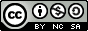## Monday, September 8, 2008

### Determining Maximum Pool Sets Using Binomial Coefficients On Linux and Unix

Hey There,How's this for some light Monday reading? ;) Actually, the title sounds a lot worse than the concept or its application. In fact, if you read the equation in its "native form," above, it looks like gibberish, unless you're really into mathematics or engineering ;) For our purposes, we'll reduce it to something that looks like regular mathematics (which it is) and tie it back into our series of posts from last week. This is what the derivation looks like in English, assuming n=4 and k=3 (no math involved ;). In fact, if you understand the concept of deriving factorials, this is really fairly basic:

The binomial coefficient of 4 over 3 is derived, in steps, by:

a. the factorial of 4 divided by the factorial of 3 times the factorial of 4 minus 3 (Complete statement)
b. 24 divided by 6 times 1.
c. 24 divided by 6.
d. 4

Maybe it's just as confusing. I can't tell ;)

Our objective in this whole set of exercises, again is: Given a Number Pool of "x through y," create the maximum possible Fixed List Length variations of our Fixed List that contain some variation of our Guaranteed Combination, without any duplication (i.e. 1, 2, 3 is equal to 2, 3, 1 and would only count as one match), and return the results. Please check out the original post on Number Pools And Guaranteed Combinations Within Fixed Lists for definitions of the different parts of our Objective.

On its face, this post doesn't seem to fit into what we've been doing lately, but (I promise) it will actually make a difference in the end :)

The equation, shown above (and in plain numerals and English below ;) has many practical applications. For our case (which I believe is what it's most commonly used for) we're going to use it to determine how many unique Y-length sets (or lists) we can derive from a X-length pool (or larger list). To put this into ordinary, and manageable perspective, you could use this equation to determine how many unique sets of 3 numbers you could find in a list of 6 numbers (1, 2, 3, 4, 5, and 6). The answer would be 20 and you could prove this to yourself easily (without mathematics) by just writing out the unique combinations on paper. Something like this, except with no computer or web browser involved ;)

123 124 125 126 = 4
134 135 136 = 7
145 146 = 9
156 = 10
234 235 236 = 13
245 246 = 15
256 = 16
345 346 = 18
356 = 19
456 = 20

We'll be using this equation, to check and ensure that we have the smallest reduced set of Fixed List Length variations of our Fixed List that contain some variation of our Guaranteed Combination, when we think we're finally finished. If we've done our job well, the numbers should match. If we haven't, well... it'll really all be my fault and I'll be sure to give myself a good mental lashing ;)

For the meantime, I've attached a simple Perl script, which should work on most distro's of Linux and/or Unix, to the bottom of this post so that you can check out the power of binomial coefficient mangling and, perhaps, put it to some good use (although we, in no way, endorse this as a method to improve your odds at gambling... So little time and so many disclaimers ;)

You can run the Perl script simply, without arguments, as it will prompt you for them (unless you retool it yourself, which is totally cool, if you want to), like so:

host # ./binco.pl
Enter Larger Pool Number: 6
Enter Smaller Set Number: 3
Binomial Coefficient of 6 over 3 = 20

Cheers,`#!/usr/bin/perl# # binco.pl - More fun with numbers :)## 2008 - Mike Golvach - eggi@comcast.net## Creative Commons Attribution-Noncommercial-Share Alike 3.0 United States License#print "Enter Larger Pool Number: ";chomp(\$x=(<STDIN>));print "Enter Smaller Set Number: ";chomp(\$y=(<STDIN>));\$z=\$x - \$y;\$fact1 = fact(\$x);\$fact2 = fact(\$y);\$fact3 = fact(\$z);\$fact4 = \$fact3 * \$fact2;\$binco = \$fact1 / \$fact4;print "Binomial Coefficient of \$x over \$y = \$binco\n";sub fact        {        my \$number = @_;        \$fact = \$number;        for (\$factless1 = \$number -1; \$factless1 > 1; \$factless1--)     {                \$fact *= \$factless1;        }        return \$fact;}`